Home > ACC7 > Chapter cc38 > Lesson cc38.3.1 > Problem8-126

8-126.
1. Simplify and solve each equation below for x. Show your work and check your answer, if possible. Homework Help ✎

1. 24 = 3x + 3

2. 2(x − 6) = y − 14

3. 3(2x − 3) = 4x − 5

4.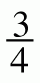y = 2x − 6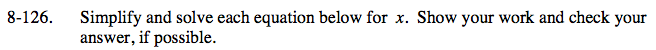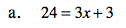Begin isolating x by subtracting 3 from both sides.
24 = 3x + 3
−3 −3
21 = 3x

Divide both sides by 3.

$\frac{21}{3}=\frac{3{\it x}}{3}$

x = 7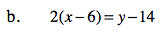Begin by distributing.

2(x) − 2(6) = y − 14
2x − 12 = y − 14
Add 12 to both sides.

2x = y − 2

$\text{Multiply by }\frac{1}{2} \text{ on each side.}$

${\it x} = \frac{1}{2}{\it y}-1$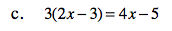Follow the methods in parts (a) and (b).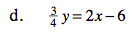Review the steps in part (b).

${\it x} = \frac{3}{8}{\it y}+3$